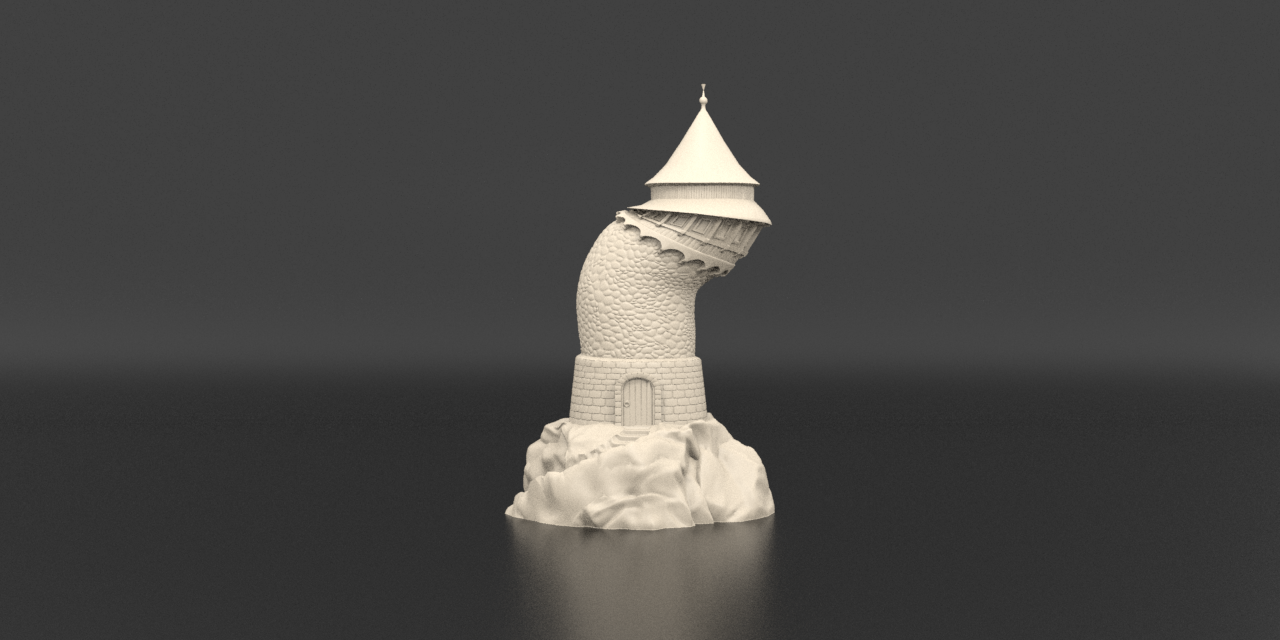# Model reduction for the material point method via an implicit neural representation of the deformation map

Journal of Computational Physics

Peter Yichen Chen, Columbia University

Maurizio Chiaramonte, Meta Reality Labs Research

Eitan Grinspun, University of Toronto, Columbia University

Kevin Carlberg, Meta Reality Labs ResearchThe reduced-order elasticity simulation contains 3 million particles, runs in real-time, and is an order-of-magnitude faster than its full-order MPM counterpart.

## Abstract

This work proposes a model-reduction approach for the material point method on nonlinear manifolds. Our technique approximates the kinematics by approximating the deformation map using an implicit neural representation that restricts deformation trajectories to reside on a low-dimensional manifold. By explicitly approximating the deformation map, its spatiotemporal gradients--in particular the deformation gradient and the velocity--can be computed via analytical differentiation. In contrast to typical model-reduction techniques that construct a linear or nonlinear manifold to approximate the (finite number of) degrees of freedom characterizing a given spatial discretization, the use of an implicit neural representation enables the proposed method to approximate the continuous deformation map. This allows the kinematic approximation to remain agnostic to the discretization. Consequently, the technique supports dynamic discretizations--including resolution changes--during the course of the online reduced-order-model simulation.

To generate dynamics for the generalized coordinates, we propose a family of projection techniques. At each time step, these techniques: (1) Calculate full-space kinematics at quadrature points, (2) Calculate the full-space dynamics for a subset of `sample' material points, and (3) Calculate the reduced-space dynamics by projecting the updated full-space position and velocity onto the low-dimensional manifold and tangent space, respectively. We achieve significant computational speedup via hyper-reduction that ensures all three steps execute on only a small subset of the problem's spatial domain. Large-scale numerical examples with millions of material points illustrate the method's ability to gain an order of magnitude computational-cost saving--indeed real-time simulations--with negligible errors.